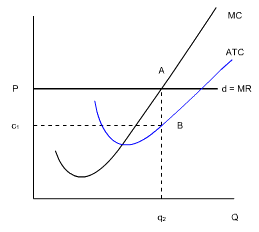### Seeing is believing!

Before you order, simply sign up for a free user account and in seconds you'll be experiencing the best in CFA exam preparation.

##### Subject 7. Breakeven Analysis and Shutdown Decision

For a price taker (a firm in a perfectly competitive market):

Profit = Total Revenue - Total Cost = (Price - Average Total Cost) x Quantity

However, maximum profit is not always a positive economic profit. In the short run, the firm might break even (making a normal profit), make an economic profit, or incur an economic loss.

1. If the price equals the minimum average total cost, the firm breaks even and makes a normal profit. A normal profit is a zero economic profit. In this case total revenue = total cost. The minimum ATC point is often referred to as the breakeven point.

2. If the price is higher than the minimum average total cost:c1BAP indicates the economic profit being made by this firm. The firm is making a positive economic profit since the price per unit exceeds the ATC per unit and the total revenue exceeds the total costs.

3. What would happen to profits if the price fell to below the ATC curve?The firm therefore will produce q1 units of output, as shown where MC = MR. At q1, the firm can only charge P per unit, and yet the ATC per unit is higher, at c2. This means that the firm is making a total economic loss equal to PBAc2, or the distance of c2 to P per unit.

If the firm's current sales revenues can cover its variable cost, and the firm anticipates that the lower market price is temporary, it will continue to operate and will face short-run economic losses. It will produce the quantity at which MC = P. This option is better than "shut down" since the firm is able to cover its variable costs and pay some of its fixed costs. If it were to shut down, the firm would lose the entire amount of its fixed costs.The shutdown point is the output and price at which the firm just covers its total variable cost.

• This point is where average variable cost is at its minimum.
• It is also the point at which the marginal cost curve crosses the average variable cost curve.
• At the shutdown point, the firm is indifferent between producing and shutting down temporarily. It incurs a loss equal to total fixed cost from either action.

If the market price is below the firm's average variable cost, a temporary shutdown is preferable to short-run operation. If the firm continues to operate, operating losses merely add to losses resulting from the firm's fixed costs. Shutdown will reduce losses.Summary

A firm should stay in the market:

• In the short run, if TR >= TVC (shutdown point), or
• In the long run, if TR >= TC (breakeven point).

Would the decision be different if the firm was operating under perfect competition or if it was a monopoly? The answer is no!

User Comment
achu In loss case, hopefully the ABPc2 area is LESS than the normal profit of the owner. If so, he/she may endure it for the short run. If person can't even make normal profit, it's truly an absolute money loser...
Lamkerst Yeah... If there is no normal profit, investors are better off investing elsewhere.
Abhinav3 This is so confusing, not possible to understand with just one or two reading..
abhishekanand A simple explanation is:
Step 1. Try to maximise profit, keep in mind that maximum profit could still be a loss. Hence maximise profit is equivalent to minimise losses if the best case is loss.

As explained in previous section, the quantity to accomplish step 1 will be when MR=MC.

Step 2. Now that you know the best you can do, see if you are making any profit when you are doing your best. Profit = Total Revenue - Total Costs = P*Q - ATC*Q = Q*(P-ATC).

So you see that (P-ATC) at Q where MR=MC will determine how much economic profit you can make in the best case.